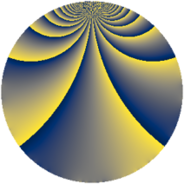# Properties

 Label 1323.2.gLevel $1323$ Weight $2$ Character orbit 1323.g Rep. character $\chi_{1323}(361,\cdot)$ Character field $\Q(\zeta_{3})$ Dimension $72$ Newform subspaces $8$ Sturm bound $336$ Trace bound $5$

# Related objects

## Defining parameters

 Level: $$N$$ $$=$$ $$1323 = 3^{3} \cdot 7^{2}$$ Weight: $$k$$ $$=$$ $$2$$ Character orbit: $$[\chi]$$ $$=$$ 1323.g (of order $$3$$ and degree $$2$$) Character conductor: $$\operatorname{cond}(\chi)$$ $$=$$ $$63$$ Character field: $$\Q(\zeta_{3})$$ Newform subspaces: $$8$$ Sturm bound: $$336$$ Trace bound: $$5$$ Distinguishing $$T_p$$: $$2$$, $$5$$

## Dimensions

The following table gives the dimensions of various subspaces of $$M_{2}(1323, [\chi])$$.

Total New Old
Modular forms 384 88 296
Cusp forms 288 72 216
Eisenstein series 96 16 80

## Trace form

 $$72q + q^{2} - 33q^{4} - 10q^{5} + 12q^{8} + O(q^{10})$$ $$72q + q^{2} - 33q^{4} - 10q^{5} + 12q^{8} + 6q^{10} + 6q^{11} + 3q^{13} - 27q^{16} + 9q^{17} + 4q^{20} + 8q^{23} + 42q^{25} + 16q^{26} + 18q^{29} + 3q^{31} - 41q^{32} + 3q^{37} - 38q^{38} - 12q^{40} + 10q^{41} + 11q^{44} - 12q^{46} + 27q^{47} + 45q^{50} - 30q^{52} - 16q^{53} + 6q^{55} - 18q^{58} + 30q^{59} - 12q^{62} + 12q^{64} - 30q^{65} + 6q^{67} - 60q^{68} - 6q^{71} - 12q^{73} + 82q^{74} - 6q^{76} + 18q^{79} + 19q^{80} + 18q^{83} + 3q^{85} + 50q^{86} + 18q^{88} + 41q^{89} + 52q^{92} + 3q^{94} + 17q^{95} + 3q^{97} + O(q^{100})$$

## Decomposition of $$S_{2}^{\mathrm{new}}(1323, [\chi])$$ into newform subspaces

Label Dim. $$A$$ Field CM Traces $q$-expansion
$$a_2$$ $$a_3$$ $$a_5$$ $$a_7$$
1323.2.g.a $$2$$ $$10.564$$ $$\Q(\sqrt{-3})$$ None $$1$$ $$0$$ $$-2$$ $$0$$ $$q+(1-\zeta_{6})q^{2}+\zeta_{6}q^{4}-q^{5}+3q^{8}+\cdots$$
1323.2.g.b $$6$$ $$10.564$$ 6.0.309123.1 None $$-1$$ $$0$$ $$-10$$ $$0$$ $$q+(\beta _{1}-\beta _{5})q^{2}+(-1+\beta _{2}+\beta _{4}+\beta _{5})q^{4}+\cdots$$
1323.2.g.c $$6$$ $$10.564$$ 6.0.309123.1 None $$-1$$ $$0$$ $$10$$ $$0$$ $$q+(\beta _{1}-\beta _{5})q^{2}+(-1+\beta _{2}+\beta _{4}+\beta _{5})q^{4}+\cdots$$
1323.2.g.d $$6$$ $$10.564$$ $$\Q(\zeta_{18})$$ None $$3$$ $$0$$ $$-6$$ $$0$$ $$q+(\zeta_{18}-\zeta_{18}^{3}-\zeta_{18}^{4}+\zeta_{18}^{5})q^{2}+\cdots$$
1323.2.g.e $$6$$ $$10.564$$ $$\Q(\zeta_{18})$$ None $$3$$ $$0$$ $$6$$ $$0$$ $$q+(\zeta_{18}-\zeta_{18}^{3}-\zeta_{18}^{4}+\zeta_{18}^{5})q^{2}+\cdots$$
1323.2.g.f $$10$$ $$10.564$$ 10.0.$$\cdots$$.1 None $$-2$$ $$0$$ $$-8$$ $$0$$ $$q-\beta _{1}q^{2}+(-\beta _{3}+\beta _{6}+\beta _{7})q^{4}+(-1+\cdots)q^{5}+\cdots$$
1323.2.g.g $$12$$ $$10.564$$ $$\mathbb{Q}[x]/(x^{12} - \cdots)$$ None $$2$$ $$0$$ $$0$$ $$0$$ $$q+\beta _{6}q^{2}+(-\beta _{1}+\beta _{4}-\beta _{5}+\beta _{6}-\beta _{7}+\cdots)q^{4}+\cdots$$
1323.2.g.h $$24$$ $$10.564$$ None $$-4$$ $$0$$ $$0$$ $$0$$

## Decomposition of $$S_{2}^{\mathrm{old}}(1323, [\chi])$$ into lower level spaces

$$S_{2}^{\mathrm{old}}(1323, [\chi]) \cong$$ $$S_{2}^{\mathrm{new}}(63, [\chi])$$$$^{\oplus 4}$$$$\oplus$$$$S_{2}^{\mathrm{new}}(189, [\chi])$$$$^{\oplus 2}$$$$\oplus$$$$S_{2}^{\mathrm{new}}(441, [\chi])$$$$^{\oplus 2}$$